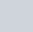The probability of an impossible event is
Question:

The probability of an impossible event is

(a) 0

(b) 1

(c) 1/2

(d) non-existent

Solution:

GIVEN: 4 options of probability of some events

TO FIND: Which of the given options is the probability of impossible event?

We know that, probability of an impossible event is 0.

Hence the correct answer is option